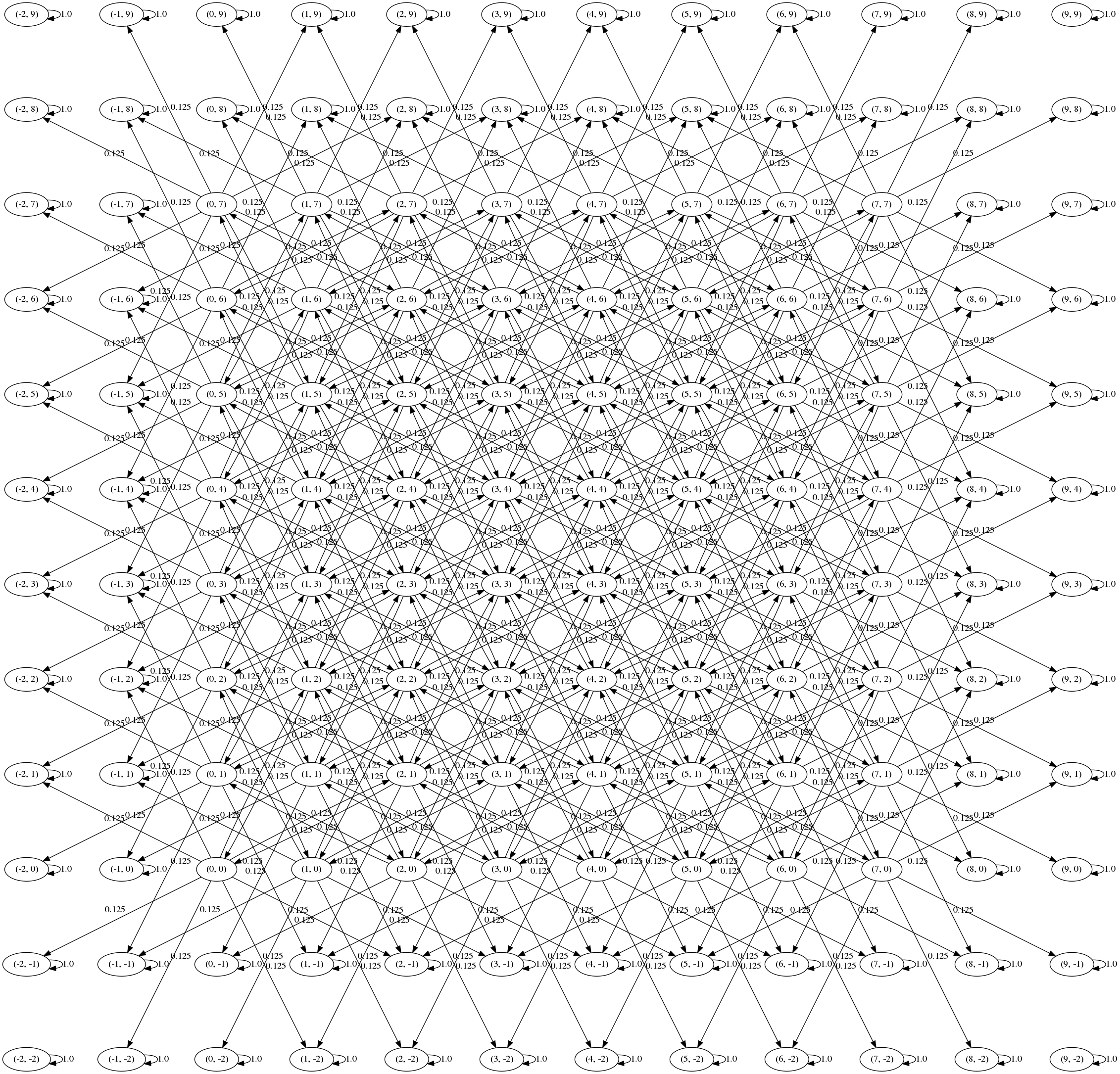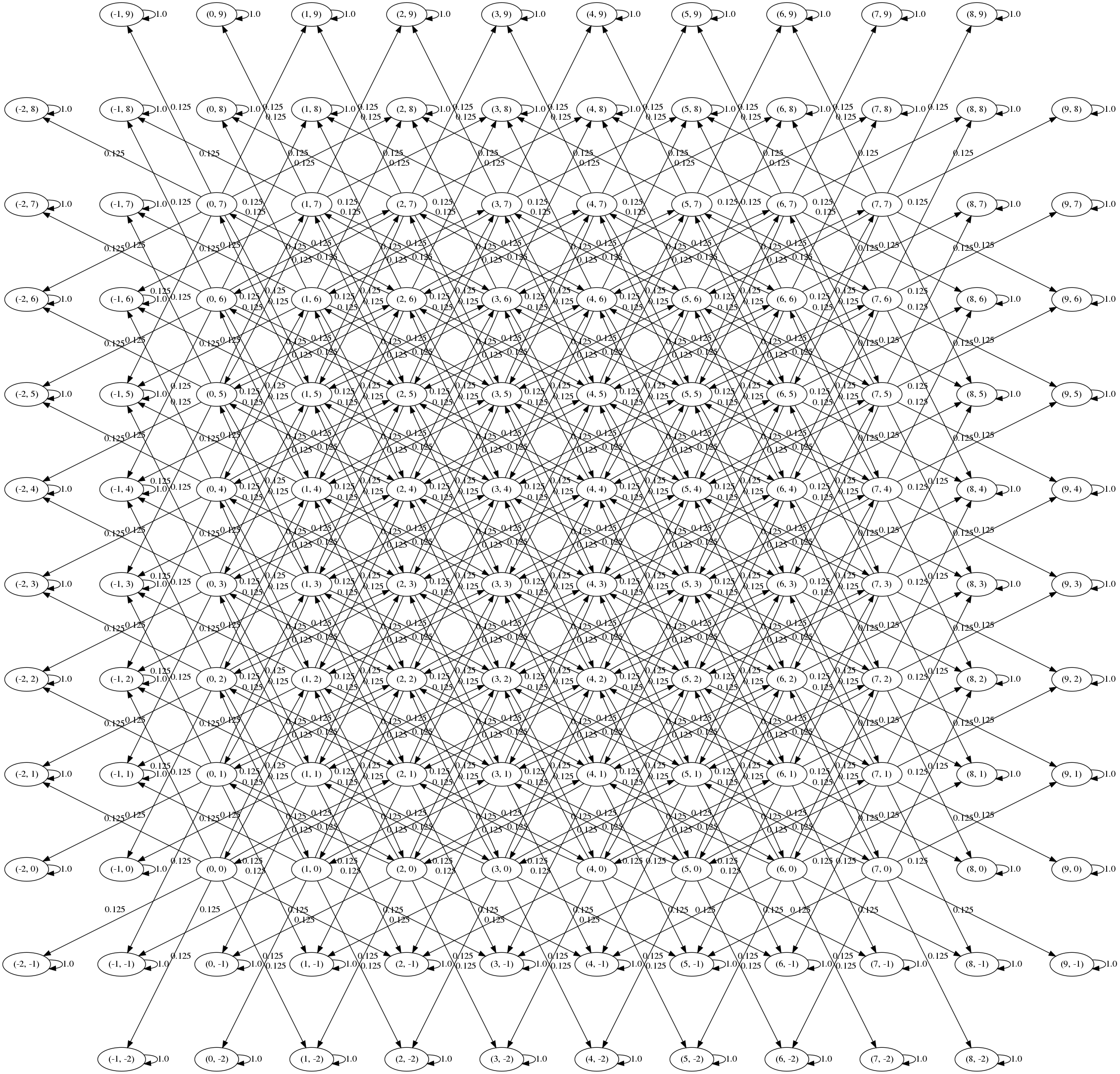Here is a well-known interview/code golf question: a knight is placed on a chess board. The knight chooses from its 8 possible moves uniformly at random. When it steps off the board it doesn’t move anymore. What is the probability that the knight is still on the board after $$n$$ steps?

We could calculate this directly but it’s more interesting to frame it as a Markov chain.

## Calculation using the transition matrix

Model the chess board as the tuples $$\{ (r, c) \mid 0 \leq i, j \leq 7 \}$$.

Here are the valid moves and a helper function to check if a move $$(r,c) \rightarrow (u,v)$$ is valid and if a cell is on the usual $$8 \times 8$$ chessboard:

The valid states are all the on-board positions plus the immediate off-board positions:

Now we can set up the transition matrix.

We can visualise the transition graph using graphviz (full code here):Oops! The corners aren’t connected to anything so we have 5 communicating classes (the 4 corners plus the rest). We never reach these nodes from any of the starting positions so we can get rid of them:

Here’s the new transition graph:Intuitively, the knights problem is symmetric, and this graph is symmetric, so it’s likely that we’ve set things up correctly.

Let $$X_0$$, $$X_1$$, $$\ldots$$, $$X_n$$ be the positions of the knight. Then then probability of the knight moving from state $$i$$ to $$j$$ in $$n$$ steps is

$P(X_n = j \mid X_0 = i) = (P^n)_{i,j}$

So the probability of being on the board after $$n$$ steps, starting from $$i$$, will be

$\sum_{k \in \mathcal{B}} (P^n)_{i,k}$

where $$\mathcal{B}$$ is the set of on-board states. This is easy to calculate using Numpy:

For this case we get probability $$0.35565185546875$$.

Here are a few more calculations:

start: (0, 0)	n: 0	Pr(on board): 1.0
start: (3, 3)	n: 1	Pr(on board): 1.0
start: (0, 0)	n: 1	Pr(on board): 0.25
start: (3, 3)	n: 4	Pr(on board): 0.48291015625
start: (3, 3)	n: 5	Pr(on board): 0.35565185546875
start: (3, 3)	n: 100	Pr(on board): 5.730392258771815e-13


It’s always good to do a quick Monte Carlo simulation to sanity check our results:

The estimate is fairly close to the value we got from taking power of the transition matrix:

pr on board from (3,3) after 5 steps: 0.3554605


## Absorbing states

An absorbing state of a Markov chain is a state that, once entered, cannot be left. In our problem the absorbing states are precisely the off-board states.

A natural question is: given a starting location, how many steps (on average) will it take the knight to step off the board?

With a bit of matrix algebra we can get this from the transition matrix $$\boldsymbol{P}$$. Partition $$\boldsymbol{P}$$ by the state type: let $$\boldsymbol{Q}$$ be the transitions of transient states (here, these are the on-board states to other on-board states); let $$\boldsymbol{R}$$ be transitions from transient states to absorbing states (on-board to off-board); and let $$\boldsymbol{I}$$ be the identity matrix (transitions of the absorbing states). Then $$\boldsymbol{P}$$ can be written in block-matrix form:

$\boldsymbol{P}= \left( \begin{array}{c|c} \boldsymbol{Q} & \boldsymbol{R} \\ \hline \boldsymbol{0} & \boldsymbol{I} \end{array} \right)$

We can calculate powers of $$\boldsymbol{P}$$:

$\boldsymbol{P}^2= \left( \begin{array}{c|c} \boldsymbol{Q} & \boldsymbol{R} \\ \hline \boldsymbol{0} & \boldsymbol{I} \end{array} \right) \left( \begin{array}{c|c} \boldsymbol{Q} & \boldsymbol{R} \\ \hline \boldsymbol{0} & \boldsymbol{I} \end{array} \right) = \left( \begin{array}{c|c} \boldsymbol{Q}^2 & (\boldsymbol{I} + \boldsymbol{Q})\boldsymbol{R} \\ \hline \boldsymbol{0} & \boldsymbol{I} \end{array} \right)$ $\boldsymbol{P}^3= \left( \begin{array}{c|c} \boldsymbol{Q}^3 & (\boldsymbol{I} + \boldsymbol{Q} + \boldsymbol{Q}^2)\boldsymbol{R} \\ \hline \boldsymbol{0} & \boldsymbol{I} \end{array} \right)$

In general:

$\boldsymbol{P}^n= \left( \begin{array}{c|c} \boldsymbol{Q}^n & (\boldsymbol{I} + \boldsymbol{Q} + \cdots + \boldsymbol{Q}^{n-1})\boldsymbol{R} \\ \hline \boldsymbol{0} & \boldsymbol{I} \end{array} \right)$

We want to calculate $$\lim_{n \rightarrow \infty} \boldsymbol{P}^n$$ since this will tell us the long-term probability of moving from one state to another. In particular, the top-right block will tell us the long-term probability of moving from a transient state to an absorbing state.

Here is a handy result from matrix algebra:

Lemma. Let $$\boldsymbol{A}$$ be a square matrix with the property that $$\boldsymbol{A}^n \rightarrow \mathbf{0}$$ as $$n \rightarrow \infty$$. Then $$\sum_{n=0}^\infty = (\boldsymbol{I} - \boldsymbol{A})^{-1}.$$

Applying this to the block form gives:

\begin{align*} \lim_{n \rightarrow \infty} \boldsymbol{P}^n &= \left( \begin{array}{c|c} \boldsymbol{Q}^n & (\boldsymbol{I} + \boldsymbol{Q} + \cdots + \boldsymbol{Q}^{n-1})\boldsymbol{R} \\ \hline \boldsymbol{0} & \boldsymbol{I} \end{array} \right) \\ &= \left( \begin{array}{c|c} \lim_{n \rightarrow \infty} \boldsymbol{Q}^n & \lim_{n \rightarrow \infty} (\boldsymbol{I} + \boldsymbol{Q} + \cdots + \boldsymbol{Q}^{n-1})\boldsymbol{R} \\ \hline \boldsymbol{0} & \boldsymbol{I} \end{array} \right) \\ &= \left( \begin{array}{c|c} \mathbf{0} & (\boldsymbol{I} - \boldsymbol{Q})^{-1}\boldsymbol{R} \\ \hline \boldsymbol{0} & \boldsymbol{I} \end{array} \right) \end{align*}

where $$\lim_{n \rightarrow \infty} \boldsymbol{Q}^n = 0$$ since all of the entries in $$\boldsymbol{Q}$$ are transient.

The top-right corner also contains the fundamental matrix as defined in the following theorem:

Theorem Consider an absorbing Markov chain with $$t$$ transient states. Let $$\boldsymbol{F}$$ be a $$t \times t$$ matrix indexed by the transient states, where $$\boldsymbol{F}_{i,j}$$ is the expected number of visits to $$j$$ given that the chain starts in $$i$$. Then $$\boldsymbol{F} = (\boldsymbol{I} - \boldsymbol{Q})^{-1}.$$

Taking the row sums of $$\boldsymbol{F}$$ gives the expected number of steps $$a_i$$ starting from state $$i$$ until absorption (i.e. we count the number of visits to each transient state before eventual absorption):

$a_i = \sum_{k} \boldsymbol{F}_{i,k}$

Back in our Python code, we can rearrange the states vector so that the transition matrix is appropriately partitioned. Taking the $$\boldsymbol{Q}$$ matrix is very quick using Numpy’s slicing notation:

Again, compare to a Monte Carlo simulation to verify that the numbers are correct:

start: (0, 0)	Avg nr steps to absorb (MC): 1.9527606
start: (0, 0)	Avg nr steps (F matrix):     1.9525249995183136

start: (3, 3)	Avg nr steps to absorb (MC): 5.4187947
start: (3, 3)	Avg nr steps (F matrix):     5.417750460813215


So, on average, if we start in the corner $$(0,0)$$ we will step off the board after $$1.95$$ steps; if we start in the centre at $$(3,3)$$ we will step off the board after $$5.41$$ steps.